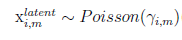# Discrete latent variables Poisson across individuals

So what I love about pyro is the ability to marginalize out discrete variables (when using for example NUTS). However, I can’t get it in the specific format I am interested in… This is the model structure:I have for each individual m latent variables, the mean of this latent variable can vary across individuals and is known. The latent variables are assumed to be Poisson distributed. I know enumeration is not possible for the Poisson variable as the distribution is unbounded. Yet for research purposes, I would like to stick to NUTS and thus I need to find a way how I could still implement this structure. My first instinct was to convert the Poisson to dist.categorical and thus disregard the extreme values that have a low probability and re-scale the other probabilities such that it still sums up to one.

Unfortunately, I got an error about tensor shapes so I added shapes. Below you can find a simplified version of my model. The model below runs however for each individual it now has the same mean and it is seen as a prior rather than a known constant (I think?). (Normally p_poisson known as gamma in the equation is calculated before model_pyro_forum_pyro is calculated).

So how could I achieve this? and is it possible to do parallel enumeration in this case?

``````def model_pyro_forum_pyro(x_latent_mean, y):
n = x_latent_mean.shape
m = x_latent_mean.shape
max_extreme_value = 15
a = np.zeros(shape=(max_extreme_value, n, m))
for i in range(0, max_extreme_value):
a[i, :, :] = poisson.pmf(i, x_latent_mean)
a = a.transpose(1,2,0)

p_poisson = Variable(torch.Tensor(a))

alpha = pyro.sample('alpha', dist.Normal(0.0, 1.0))

with pyro.plate('latent_value', m):
latent_coef = pyro.sample('latent_coef',
dist.Normal(0.0, 0.25))

with pyro.plate('data'):
x_latent = pyro.sample(f"x_latent", dist.Categorical(p_poisson[0, :, :]),
infer={"enumerate": "sequential"})
y_loc = alpha + (x_latent * latent_coef).sum(dim=-1)
pyro.sample('y', dist.Bernoulli(logits=y_loc), obs=y)
``````

Hi @Helena.H,

It looks like there is a conflicting dim in your `x_latent`. IIUC `x_latent` should have shape `(len(data), m)`. Maybe nest your plates and specify an explicit `dim` kwarg?

``````latent_plate = pyro.plate('latent_value', m, dim=-1)
with latent_plate:
latent_coef = ...
with pyro.plate('data', len(y), dim=-2):
with latent_plate:
x_latent = ...
y_loc = ...
pyro.sample('y', ..., obs=y.unsqueeze(-2))
``````

However I don’t believe you can enumerate here, because there is a downstream coupling of the `x_latent` outside of the latent plate: this violates the assumptions of parallel enumeration. I think to correct your approach, you would need to separately enumerate each poisson variable, which grows as the cartesian product of your enumeration domains.

I think in your case I would try to look for some sort of separability propert in the `y` likelihood so that you could move it inside the `x_latent` plate; sometimes this works for `Binomial` or similar likelihoods. Then you could parallel enumerate.

Good luck!

1 Like

I tried a few things yet the structure you proposed above resulted in the following error:
Allowed dims: -2
Actual shape: (6000, 6000)
Try adding shape assertions for your model’s sample values and distribution parameters.
Where len(y) was 6000.
The shapes are denoted as follows:
latent_coef dist 2 |
value 2 |
log_prob 2 |
data dist |
value 6000 |
log_prob |
x_latent dist 6000 2 |
value 6000 2 |
log_prob 6000 2 |
y dist 6000 6000 |
value 1 6000 |
log_prob 6000 6000 |

I implemented it in the following way:

``````latent_plate = pyro.plate('latent_value', m, dim=-1)
with latent_plate:
latent_coef = pyro.sample('latent_coef',
dist.Normal(0.0, 0.25))

with pyro.plate('data', len(y), dim=-2):
with latent_plate:
x_latent = pyro.sample(f"x_latent", dist.Categorical(mean_poisson),
infer={"enumerate": "sequential"})
y_loc = alpha + (x_latent * latent_coef).sum(dim=-1)
pyro.sample('y', dist.Bernoulli(logits=y_loc), obs=y.unsqueeze(-2))``````